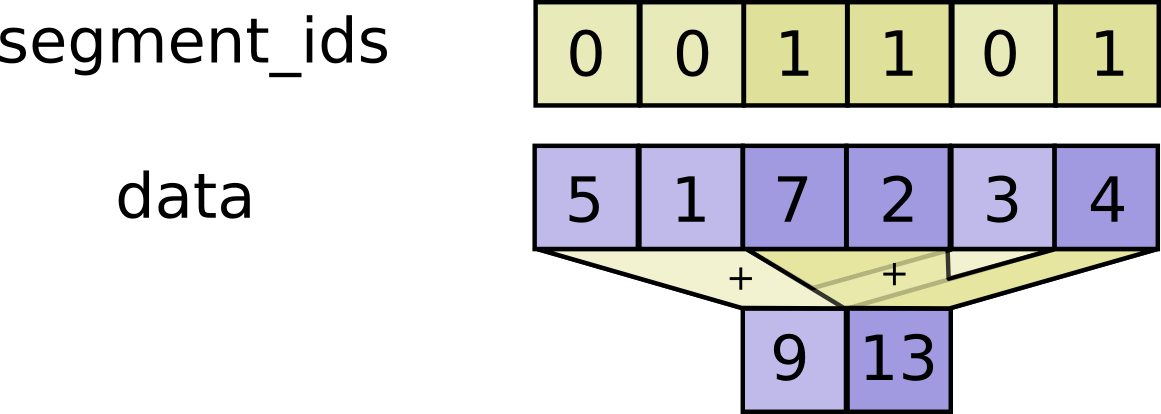Help protect the Great Barrier Reef with TensorFlow on Kaggle

# tf.math.unsorted_segment_sum

Computes the sum along segments of a tensor.

Read the section on segmentation for an explanation of segments.

Computes a tensor such that $$output[i] = \sum_{j...} data[j...]$$ where the sum is over tuples j... such that segment_ids[j...] == i. Unlike SegmentSum, segment_ids need not be sorted and need not cover all values in the full range of valid values.

If the sum is empty for a given segment ID i, output[i] = 0. If the given segment ID i is negative, the value is dropped and will not be added to the sum of the segment.

num_segments should equal the number of distinct segment IDs.c = tf.constant([[1,2,3,4], [5,6,7,8], [4,3,2,1]])
tf.unsorted_segment_sum(c, tf.constant([0, 1, 0]), num_segments=2)
# ==> [[ 5,  5, 5, 5],
#       [5,  6, 7, 8]]


data A Tensor. Must be one of the following types: float32, float64, int32, uint8, int16, int8, complex64, int64, qint8, quint8, qint32, bfloat16, uint16, complex128, half, uint32, uint64.
segment_ids A Tensor. Must be one of the following types: int32, int64. A tensor whose shape is a prefix of data.shape.
num_segments A Tensor. Must be one of the following types: int32, int64.
name A name for the operation (optional).

A Tensor. Has the same type as data.

[{ "type": "thumb-down", "id": "missingTheInformationINeed", "label":"Missing the information I need" },{ "type": "thumb-down", "id": "tooComplicatedTooManySteps", "label":"Too complicated / too many steps" },{ "type": "thumb-down", "id": "outOfDate", "label":"Out of date" },{ "type": "thumb-down", "id": "samplesCodeIssue", "label":"Samples / code issue" },{ "type": "thumb-down", "id": "otherDown", "label":"Other" }]
[{ "type": "thumb-up", "id": "easyToUnderstand", "label":"Easy to understand" },{ "type": "thumb-up", "id": "solvedMyProblem", "label":"Solved my problem" },{ "type": "thumb-up", "id": "otherUp", "label":"Other" }]